# Numerical Simulation and Anomalies Qualification Based on Ground-well Transient Electromagnetics Method

Numerical Simulation and Anomalies Qualification Based on Ground-well Transient Electromagnetics Method

Jun Zhang Baixiang Liu *Yanqing Wu Hongchun Yi

School of Geosciences and Info-Physics, Central South University, Changsha 410083, China

China Coal Technology and Engineering Group Chongqing Research Institute, Chongqing 400039, China

Key Laboratory of Metallogenic Prognosis and Geological Environment Monitoring, Ministry of Education, Central South University, Changsha 410083, China

Chongqing University, School of Resources and Environmental Sciences, Chongqing 400044, China

Corresponding Author Email:
178244944@qq.com
Page:
235-240
|
DOI:
https://doi.org/10.18280/ejee.210216
16 January 2019
|
Accepted:
18 March 2019
|
Published:
30 June 2019
| Citation

OPEN ACCESS

Abstract:

The ground-well transient electromagnetics (G-W TEM) method is an important way to enhance the resolution and depth of detection, for its signals, emitted on the ground and received in the well or tunnel, are close to anomalies. This paper attempts to detect deep, small anomalies accurately through the G-W TEM. Drawing on the 2D finite-difference time-domain (FDTD) method and the ground TEM method, several field potential models were created for plate-shape conductors and square conductors at different depths and locations. The model construction was conducted with a large fixed loop as the emitter, assuming that the medium obeys uniform distribution. The signals were observed under the ground-borehole or ground-tunnel modes, and subjected to forward modelling, with the aim to disclose the response characteristics of small anomalies to the vertical magnetic field intensity (Hz) curve and multi-track electromagnetic absorption (EA) curves. On this basis, the author put forward an interpretation method that accurately locate small anomalies based on the extreme point of the Hz curve and the intersections of the EA curves. The research results show that the FDTD method can solve the established field potential models of linear conductors in an effective and accurate manner, and output the curve response characteristics of small anomalies; under the ground-borehole mode, the longitudinal position of each anomaly can be identified, and different anomalies can be distinguished based on the extreme point of the Hz curve, and the amplitude of the TEM response curve decreases with the elapse of time; under the ground-tunnel mode, the lateral position of each anomaly can be identified, and different anomalies can be distinguished based on the intersections of EA curves, and the EA value is negatively correlated with the distance to the anomaly. To sum up, the proposed method pinpoints small anomalies based on the extreme point of the Hz curve and the intersections of the EA curves, and improves the resolution in both vertical and horizontal directions.

Keywords:

ground-well transient electromagnetics (G-W TEM), linear conductor, observation mode, curve feature

1. Introduction

With the development and utilization of deep coal resources in China, it becomes increasingly important to conduct effective exploration of deep geological conditions. The ground TEM is currently the most commonly used detection method, but its detection depth has gradually failed to meet the current exploration needs. In this context, the ground-well transient electromagnetics (G-W TEM) has been a hot spot of current research, because it uses a large fixed source loop to transmit, the well receives, and the distance from anomaly is short, so that the resolution and effective detection depth is greatly improved.

Domestic and foreign scholars have carried out more research work on this, but mostly at the theoretical research stage for now. Eaton and Hohmann , West and Ward  used the integral equation to study the anomalous G-W TEM field response characteristics under long wire sources; Yang et al.  established a three-dimensional geological model and the overlay model, to analyse the response characteristics of the square low-resistance object and overburden layers as well as the influence factors of the overlayer. Wu et al.  defined the global apparent resistivity of grounded source G-W TEM; in literature  they verified the effectiveness of G-W TEM for determining electrical interface under the condition of electrical emission source; their study in  further showed that the three-component transient response curve of G-W TEM has a good response to electrical interfaces and anomality. Xu et al.  studied the vertical contact zone and its G-W TEM response characteristics when containing low resistance bodies; they also performed forward modelling of uniform half-space and vertical fault planes , and carried out three-dimensional forward modelling of G-W TEM, summarizing the main factors affecting G-W TEM anomalies ; Meng and Pan  calculated the G-W TEM anomaly response of low-resistance plate-shaped conductors in the case of homogeneous half-space and low-resistance coating, and studied the response characteristics and laws; Meng et al.  believe that the response obtained by G-W TEM observation is the result of the interaction between the surrounding rock background field and the target anomaly field, and proposed that the earth medium will affect the observation results; Meng et al.  calculated and analysed the multi-component response of the G-W TEM, obtaining the results that the response characteristics depend on the "diffusion, attenuation, distortion" process of the transient field in the formation under different conditions and the electromagnetic field state of the observed position. Miao et al.  carried out the design and application research of mine ground-tunnel TEM detection system; Fan  used the borehole- transient electromagnetic method to carry out the test of goaf in advance detection; Li et al.  studied the TEM response curves of different aquifers and inclined water-filled faults using ground-downhole TEM; Yi  conducted research on the G-W TEM response characteristics in lower half space of the rectangular loop source. Tang et al.  studied the three-component electromagnetic response of simulated three-dimensional cuboids and spheres.

This paper attempts to detect deep, small anomalies accurately through the G-W TEM. Drawing on the 2D FDTD method and the ground TEM method, several field potential models for linear conductor were created, assuming that the medium obeys uniform distribution. The signals were observed under the ground-borehole or ground-tunnel modes. The vertical magnetic field strength Hz and EA multi-track curves were drawn, with the aim to disclose their response characteristics, and accurately locate small anomalies based on the extreme point of the Hz curve and the intersections of the EA curves. This shall provide a reliable theoretical basis for improving the resolution of TEM detection in the vertical and horizontal direction, and increasing the effective depth.

This paper consists of four parts. The first part is the background and literature review; the second part introduces the basic principle of FDTD; the third part performs the G-W TEM simulation and analyses the response characteristics; the fourth part is the conclusion.

2. Basic Principle of Finite Difference Time Domain Method

2.1 Mur absorption boundary conditions

Using x=xmax as the boundary, this paper adopts the Mur absorption boundary condition as expressed in equation (1). The derivation process is shown in .

$\left(\frac{\partial}{\partial x}-\frac{1}{c} \frac{\partial}{\partial t}\right)\left.E\right|_{x=\max }=0$        (1)

The side node difference is derived as (2):

$E_{\max }^{n+1}=E_{\max -1, k}^{n}+\frac{c \Delta t-\delta}{c \Delta t+\delta}\left[E_{\max -1, k}^{n+1}-E_{\max , k}^{n}\right]$       (2)

where, $c=1 / \sqrt{\mu_{0} \varepsilon_{0}}$ ; $\mu_{0}$ is the vacuum permeability, H. m-1; $\varepsilon_{0}$  is dielectric constant, F. m-1; E is electric field strength, V. m-1; δ is node spacing, m; t is the time step increment, s.

2.2 Incentive field source

The large fixed loop excitation device was selected. The electric field in the y direction of the uniform half-space layered medium for the loop source was calculated as shown in equation (3). The detailed derivation process can be found in literature :

\begin{align} & {{E}_{y}}=\frac{I}{\pi \sigma {{r}^{2}}}\left\{ 2{{\theta }^{2}} \right.{{z}^{2}}{{e}^{-{{\theta }^{2}}{{r}^{2}}}}+\frac{{{x}^{2}}-{{z}^{2}}}{{{r}^{2}}}(erfc(\theta z)-{{e}^{-{{\theta }^{2}}{{r}^{2}}}}) \\ & \ \ \ \ \ \ \ -\frac{2}{\sqrt{\pi }}\theta z{{e}^{-{{\theta }^{2}}{{r}^{2}}}}[1-2\theta x(1+\frac{1}{{{\theta }^{2}}{{r}^{2}}})F(\theta x)\left. ] \right\} \\\end{align}       (3)

where, $\theta=\left(\frac{\mu \sigma}{4 t}\right)^{1 / 2}$ , $r^{2}=x^{2}+z^{2}$ ; μ is permeability, H. m-1; σ is conductivity, S. m-1; r is the radius of the loop source, m; x is the side margin, m; z is depth, m; erfc is complementary error function, F(θx) is Dawson integral.

3. Numerical Simulation and Response Characteristics Analysis of Ground-well Transient Electromagnetics

Through the ground-bore and ground-tunnel observation modes the plate-shaped conductor and the square conductor were simulated, and the vertical magnetic field strength Hz and EA multi-track curves were drawn, so as to analyse and quantify the G-W TEM response characteristics.

3.1 Ground-borehole observation mode

It refers to the use of large fixed loop device for emitting on the ground with a 400 m × 400 m square coil and a current intensity of 10A. The high frequency probe is used for receiving in the borehole at the receiving point of 10m and the detection depth of 500 m (Figure 1).

## 1.png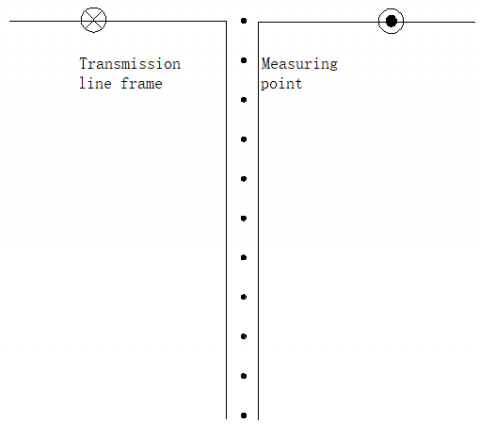Figure 1. Diagram of ground-borehole observation mode

Assuming that the earth resistivity ρground=100 Ω·m, the borehole is at the centre position (x=0). Figure 2 shows the TEM response multi-track curve of vertical magnetic field strength Hz(x)-measuring depth (y) at different time points (t1-t6, corresponding to 0.15, 0.2, 0.25, 0.3, 0.35, 0.4ms from right to left) under the ground-borehole observation mode.

Figure 2a shows an field potential model of the plate-shaped low-resistance with a thickness of 2 m, a depth of z=200 m and a resistivity of ρ1=10 Ω·m. It can be seen from the figure that the amplitude of the early TEM response curve was the maximum, and with the induced magnetic field gradually attenuating over time, the amplitude was getting smaller; for the position of the measuring line, if it’s closer to the plate-shaped conductor, there appears the maximum value of the vertical magnetic field strength Hz, corresponding to the low-resistance body at a depth of 200 m in the model; Figure 2b shows the two field potential models of the plate-shaped low-resistance with a thickness of 2 m, a depth of z=200, 300 m and a resistivity of ρ1=10 Ω·m, which indicates that the amplitude of the early TEM response curve is similar to that of a single plate-shaped low-resistance model, and the amplitude becomes smaller with time increasing; for the position of the measuring line, two extreme values appeared in the vertical magnetic induction intensity Hz, corresponding to the depth of the 200, 300 m low-resistance plate-shaped body in the model. Therefore, the G-W TME makes more obvious response to the plate-shaped low-resistance body, with a higher longitudinal resolution.

## 2.png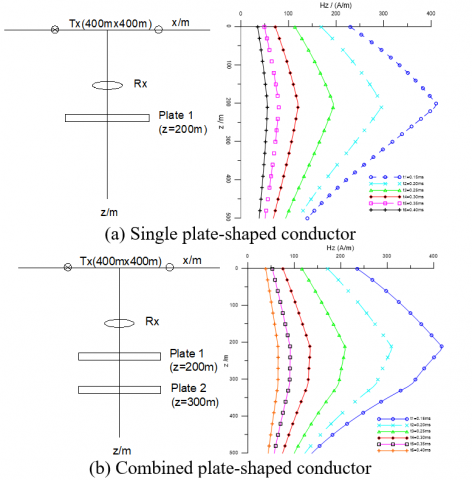Figure 2. TEM response curves of plate-shaped conductor under the ground-borehole measurement mode

3.2 Ground-tunnel observation mode

It refers to the use of large-fixed loop device for emitting on the ground, and high-frequency probe for receiving in the downhole tunnel. The transmitting and receiving parameters are consistent with the ground-borehole observation mode. Three measuring lines were arranged at Z=0, 300, 500 m, and then sequentially labelled as 1#, 2#, 3#; each measuring line had a length of 400m. Assuming that the earth resistivity ρground=100 Ω·m, the electric field model of a 2 m×2 m square low-resistance body at the position x=100 m, and depth z=400m of measuring line was created (Figure 3).

Figure 4 shows the multi-track TEM response curve of the line position (x) and the vertical magnetic field strength Hz(y) at different time points (t1-t6, the same as in the model above) under the ground-tunnel observation mode. From the perspective of the curve amplitude, the response to low resistance anomalies is not very obvious.

## 3.png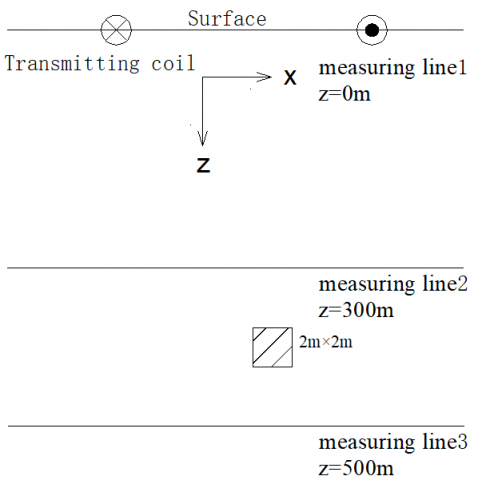Figure 3. Diagram of ground-tunnel measurement mode

## 4.png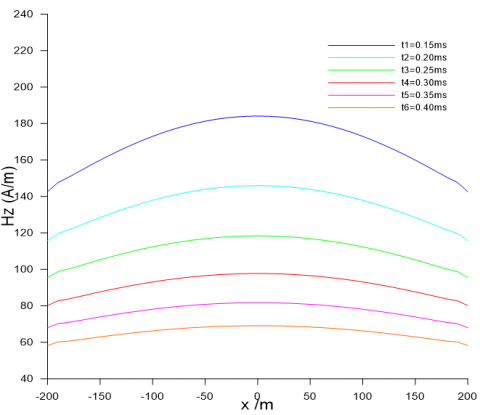Figure 4. The Hz response curve of measuring line 1

This paper attempts to improve the ability to distinguish the anomaly and realize the fine detection of the deep structure. For this, the paper draws on the research results of the amplitude difference between the electromagnetic signals in the disturbance and the intact coal seam by Greenfield and Wu , and redefines the EA calculation method in equation (4). Then, it draws the EA multi-track curve with single anomaly and different lines, as shown in Figure 5.

EA(x)=Hz(x)-Hz0(x)         (4)

where: Hz0(x)-vertical magnetic field strength measured without anomaly, A.m-1; Hz(x)-vertical magnetic field strength measured with anomalous body, A.m-1; EA(x)-difference of vertical magnetic field strength with or without anomaly, A.m-1. Therefore, the EA value indicates a low-resistance anomaly "pure response".

In Figure 5, the EA multi-track curves correspond to different time points t1-t6 from top to bottom. Figure 5a, 5b and 5c show that the three lines have a more pronounced response to the low-resistance anomaly, and the intersections of the three EA multi-track curves correspond to the positions of the low-resistance anomaly, so it can be seen that the intersection of the EA multi-track curves indicates a low-resistance anomaly. Compared with Figure 4, Figure 5a, the exact position of the anomaly can be obtained in Figure 5a, 5b, and 5c through the intersection; compared with Figure 5a, the EA value of Figure 5b and 5c increased from 6×10-3 to 3×10-1, by 2 orders of magnitude, indicating the closer the receiving probe is to the low-resistance anomaly body, then the larger the EA value is, and the higher the detection resolution is.

## 51.png(a) EA curve of 1# line (z=0m)

## 52.png(b) EA curve of 2# line (z=300m)

## 53.png(c) EA curve of 3# line (z=500m)

Figure 5. EA multiple track curves with single anomaly and different lines

In order to further study the lateral resolution of the G-W TEM, two field potential models of the 2 m×2 m low-resistance body were established at the line position x=+100m, x=-100 m, and depth z=400 m. The three lines of 1#, 2#, and 3# and other parameters are consistent with the previous two models, as shown in Figure 6.

## 6.png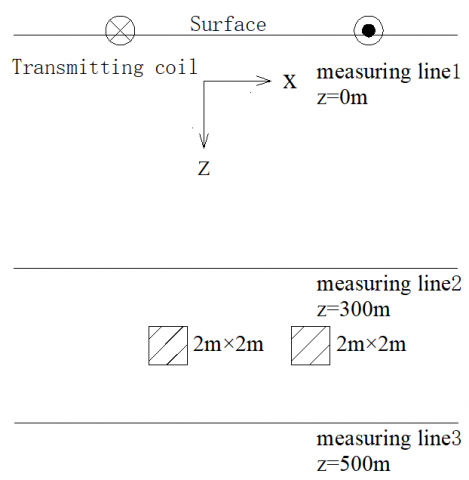Figure 6. Model with 2 low-resistivity anomaly body

Figure 7a shows the EA curve of the 1# line (z=0). Since the 1# line is far away from the low-resistance body, the curves at different times do not completely intersect, with no obvious intersection, so that the lateral position of the two low-resistance bodies cannot be accurately and clearly distinguished at a low resolution.

## 71.png(a) EA curve of 1# line (z=0)

## 72.png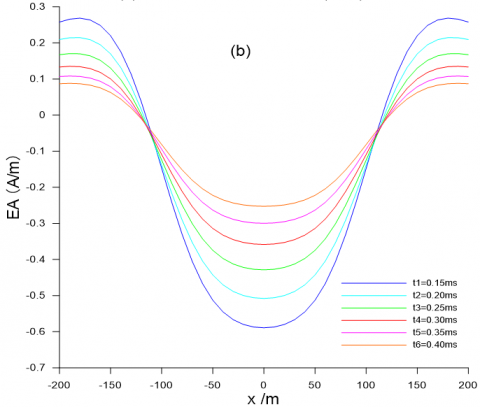(b) EA curve of 2# line (z=300m)

## 73.png(c) EA curve of 3# line (z=500m)

Figure 7. More EA multiple track curves with different lines

Figure 7b and 7c show the EA curve of the 2# line (z=300 m) and the EA curve of the 3# line (z=500 m) respectively. These the two lines are closer to the low-resistance body, so there are two obvious intersections in the two EA curves, which correspond to the lateral position (x=+100m, x=-100m) of the two low-resistance bodies in the model at a high lateral resolution.

4. Conclusions

(1) The FDTD method can solve the established field potential models of linear conductors in an effective and accurate manner, and output the curve response characteristics of small anomalies;

(2) Under the ground-borehole mode, the longitudinal position of each anomaly can be identified, and different anomalies can be distinguished based on the extreme point of the Hz curve, and the amplitude of the TEM response curve decreases with the elapse of time;

(3) Under the ground-tunnel mode, the lateral position of each anomaly can be identified, and different anomalies can be distinguished based on the intersections of EA curves, and the EA value is negatively correlated with the distance to the anomaly;

(4) Based on the extreme point of the Hz curve and the intersections of the EA curves, small anomalies can be accurately positioned, and the resolution in both vertical and horizontal directions can be improved.

Acknowledgment

The project is supported by the State Key R&D Program of the Ministry of Science and Technology of China (Grant No. 2018YFC0807805).

References

 Eaton, P.A., Hohmann, G.W. (1984). The influence of a conductive host on two-dimensional borehole transient electromagnetic responses. Geophysics, 49(7): 861-869. https://doi.org/10.1190/1.1441732

 West, R.C., Ward, S.H. (1988). The borehole transient electromagnetic response of a three-dimensional fracturezone in a conductive half-space. Geophysics, 53(11): 1469-1478. https://doi.org/10.1190/1.1442427

 Yang, H.Y., Yue, J.H., Xu, Z.Y., Zhang, H., Jiang, Z.H. (2016). Transient electromagnetic method modeling in ground-borehole model with overburden influence. Journal of Jilin University (Earth Science Edition), 46(5): 1527-1537. http://dx.chinadoi.cn/10.13278/j.cnki.jjuese.201605301

 Wu, J.J., Li, X., Zhi, Q.Q., Qi, Z.P., Guo, J.L., Deng, X.H., Zhang, J., Wang, X.C., Yang, Y. (2017). Full field apparent resistivity definition of Borehole TEM with electric source. Journal of Geophysics, 60(4): 1595-1605. https://doi.org/10.6038/cjg20170431

 Wu, J.J., Li, X., Zhi, Q.Q., Deng, X.H., Zhang, J., Wang, X.C., Yang, Y. (2017). Preliminary analysis of transient electromagnetic anomaly field response characteristics of electrical source-well. Geophysical and Geochemical Exploration, 41(1): 129-135. http://dx.chinadoi.cn/10.11720/wtyht.2017.1.20

 Wu, J.J., Li, X., Zhi, Q.Q., Qi, Z.P., Guo, J.L., Deng, X.H. (2017). Three-component response analysis of electrical source-well transient electromagnetic method. Progress in Geophysics, 32(3): 1273-1278.

 Xu, Z.Y., Yang, H.Y., Deng, J.Z., Tang, H.Z., Zhang, H., Xie, S.W., Liu, S.G. (2015). Transient electromagnetic response of underground-well under the influence of vertical contact zone. Progress in Geophysics, 30(3): 1345-1353.

 Xu, Z.Y., Yang, H.Y., Deng, J.Z., Tang, H.Z., Zhang, H. (2015). 3 Dimensions FDTD Numerical Simulation on the Down-hole TEM Field with a Loop Source. Chinese Journal of Engineering Geophysics, 12(3): 327-332. http://dx.chinadoi.cn/10.3969/j.issn.1672-7940.2015.03.009

 Xu, Z.Y., Yang, H.Y., Deng, J.Z., Tang, H.Z., Zhang, H., Liu, X.H., Zhao, H.J., Gong, J.H. (2015). Forward modeling of ground-well transient electromagnetic method based on anomalous field. Geophysical and Geochemical Exploration, 39(6): 1176-1182. http://dx.chinadoi.cn/10.11720/wtyht.2015.6.13

 Meng, Q.X., Pan H.P. (2012). Numerical simulation analysis of surface-hole TEM responses. Chinese Journal of Geophysics, 55(3): 1046-1053. http://dx.chinadoi.cn/10.6038/j.issn.0001-5733.2012.03.035

 Meng, Q.X., Pan, H.P., Niu, Z. (2014). Forward simulation of surface-borehole TEM in geological medium effect. Journal of China University of Mining & Technology (Social Science), 43(6): 1113-1119.

 Meng, Q.X., Hu, X.Y., Pan, H.P., Zhou, F. (2017). Multicomponent numerical analysis of ground-well transient electromagnetic response. Applied Geophysics, 14(1): 175-186, 192. http://dx.chinadoi.cn/10.1007/s11770-017-0600-6

 Miao, B., Jiang, Z.H., Liu, S.C. (2016). Design and application of transient electromagnetic detection system on mine surface ground and in underground mine roadway. Coal Science and Technology, 44(12): 148-153. https://doi.org/10.13199/j.cnki.cst.2016.12.026

 Fan, T. (2017). Test of detecting goaf by fixed receiving method of transient electromagnetic power source in tunnel. Journal of China Coal Society, 42(12): 3229-3238. https://doi.org/10.13225/j.cnki.jccs.2017.0450

 Li, S.C., Li, K. Zhai, M.H., Sun, H.F., Wang, S.R. (2015). Analysis of transient electromagnetic detection response law of surface-underground electrical source in mine. Journal of China Coal Society, 41(8): 2024-2032. https://doi.org/10.13225/j.cnki.jccs.2015.1928

 Yi, H.C. (2018). Study on transient electromagnetic response characteristics of ground-well. Geophysical and Geochemical Exploration, 42(5): 970-976.

 Tang, J.Q., Xi, Z.Z., Wang, H., Lei, K., Li, L. (2015). The regularity of three-component measurement responses in surface-hole TEM. Chinese Journal of Engineering Geophysics, 12(3): 315-321. http://dx.chinadoi.cn/10.3969/j.issn.1672-7940.2015.03.007

 Ge, D.B. (2002). Finite difference time domain method of electromagnetic wave. Xi'an University of Electronic Science and Technology Press, Xi'an, China.

 Mizak, N. (1992). Exploration Geophysical Electromagnetic Method. Translated, Geological Publishing House, Beijing China.

 Greenfield, R.J., Wu, S.T. (1991). Electromagnetic wave propagation in disrupted coal seams. Geophysics, 56(10): 1571-1577. http://dx.doi.org/10.1190/1.1892222